Mixed Model diagnostics

Here we take a dataset from the spatial-autocorrelation R code and use it to demonstrate some of the key approaches to mixed model diagnostics

First we load the required R packages, read in the dataset and resave the models we use as new objects

library(lme4)
library(nlme)
library(geoR)

#N.B. path will need setting

#Refit region model
model<-lmer(height~temp+(1|region),data=data)
#Refit site model
model2<-lmer(height~temp+(1|site),data=data)
#Fit null model
model.n<-lm(height~temp,data=data)

Step one. Simple Diagnostic plots of the residuals

Just like for a linear model it is important to inspect diagnostic plots of the residuals. Plotting the residuals against the fitted values facilitates the detection of heteroscedasticity, while plotting them against the explanatory variable(s) helps detect non-linearity

#Default plot of standardised residuals versus fitted
plot(model, resid(., scaled=TRUE) ~ fitted(.), abline = 0,pch=16,xlab="Fitted values",ylab="Standardised residuals")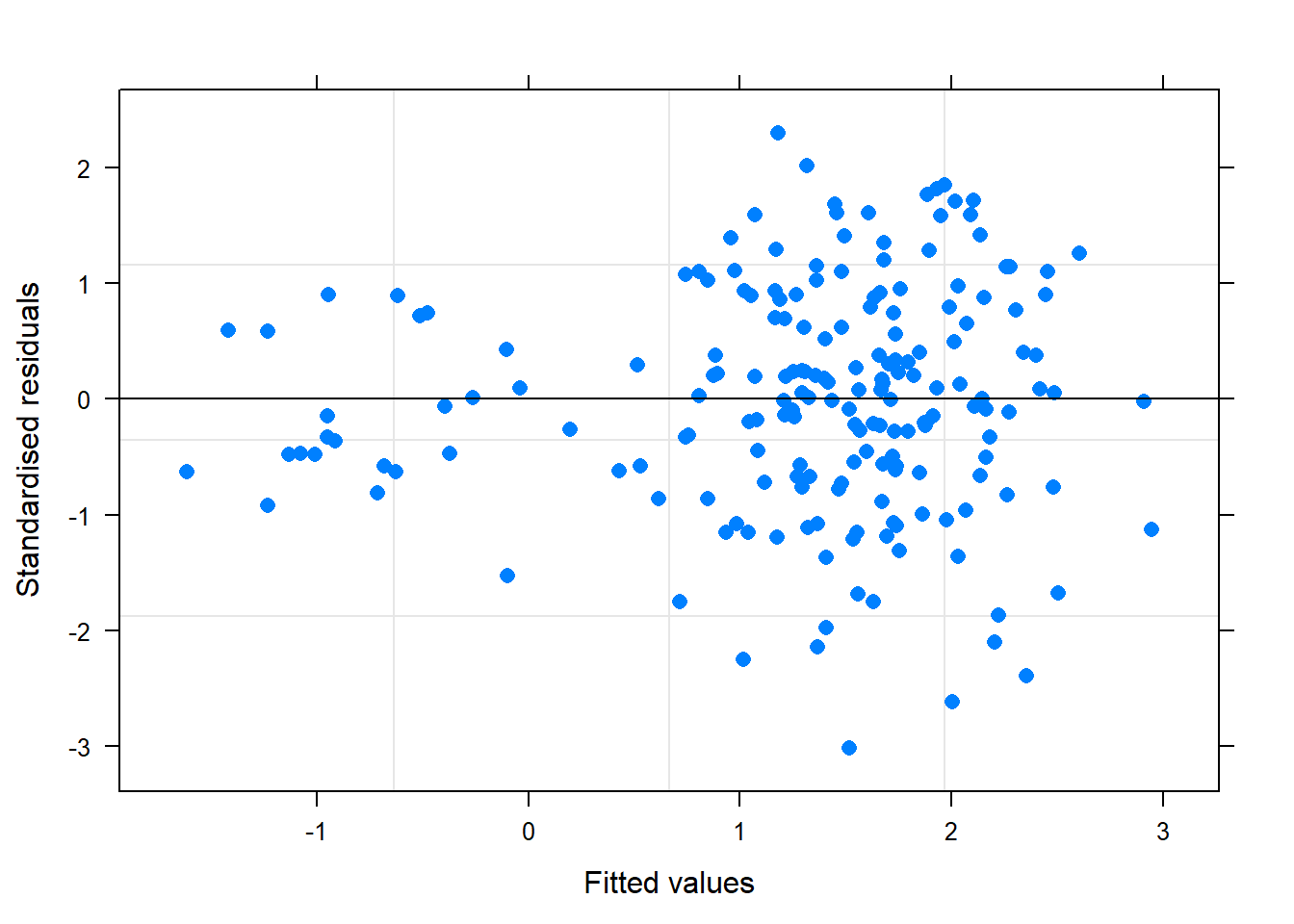#You can also colour this plot by the levels of your random effect (in our case region)
#In this case we let R pick colours
plot(model, resid(., scaled=TRUE) ~ fitted(.), abline = 0,pch=16,col=data\$region,xlab="Fitted values",ylab="Standardised residuals")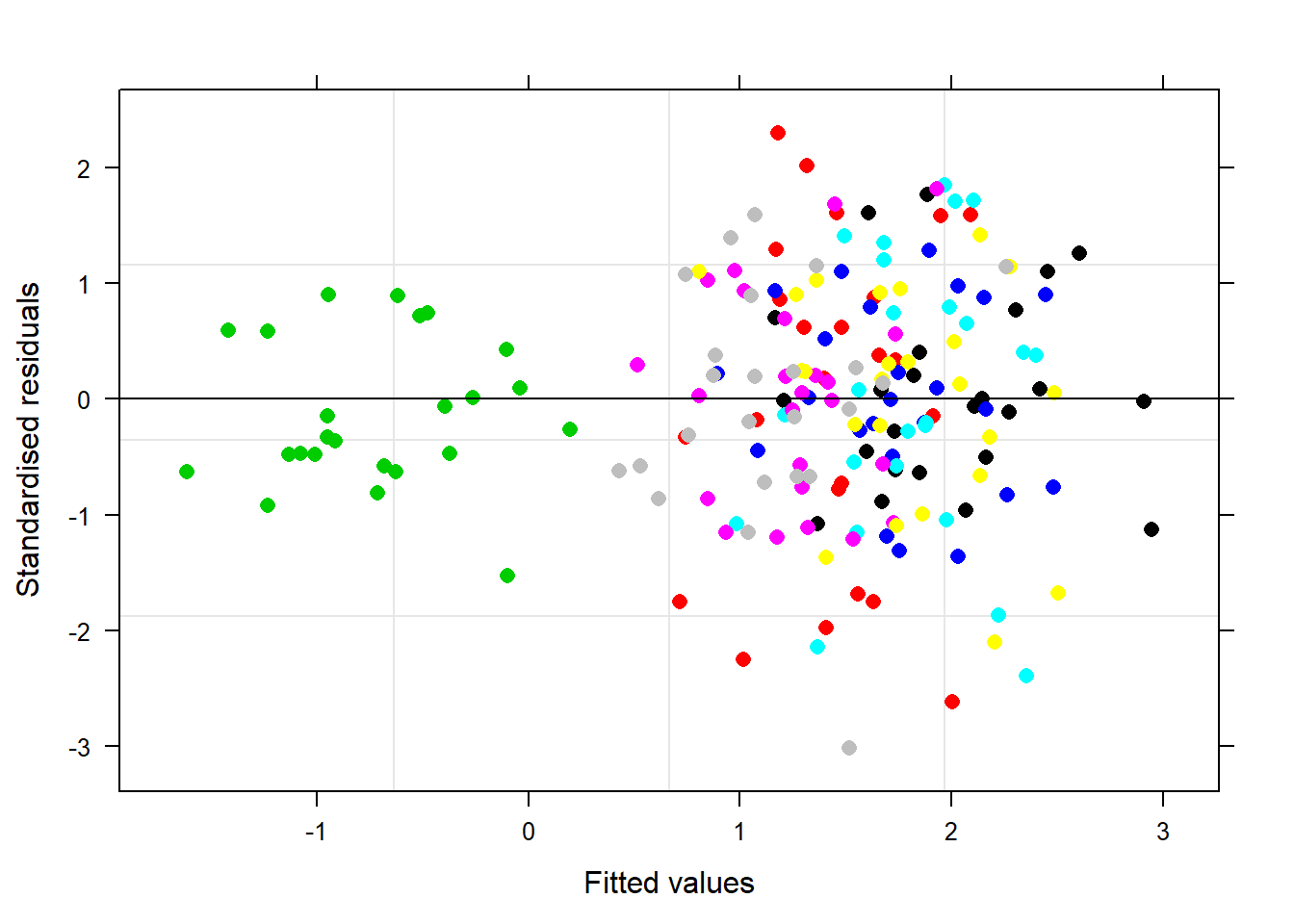#You can plot the residuals against the fitted values manually using the resid() and predict () functions
#We also demonstrate the use of user-defined colours here
cols=c("#332288", "#88CCEE", "#44AA99", "#117733", "#999933", "#DDCC77", "#CC6677","#AA4499")
plot(resid(model,type="pearson")~predict(model),pch=16,col=cols[data\$region],xlab="Fitted values",ylab="Standardised residuals")
lines(x=c(-1000,1000),y=c(0,0))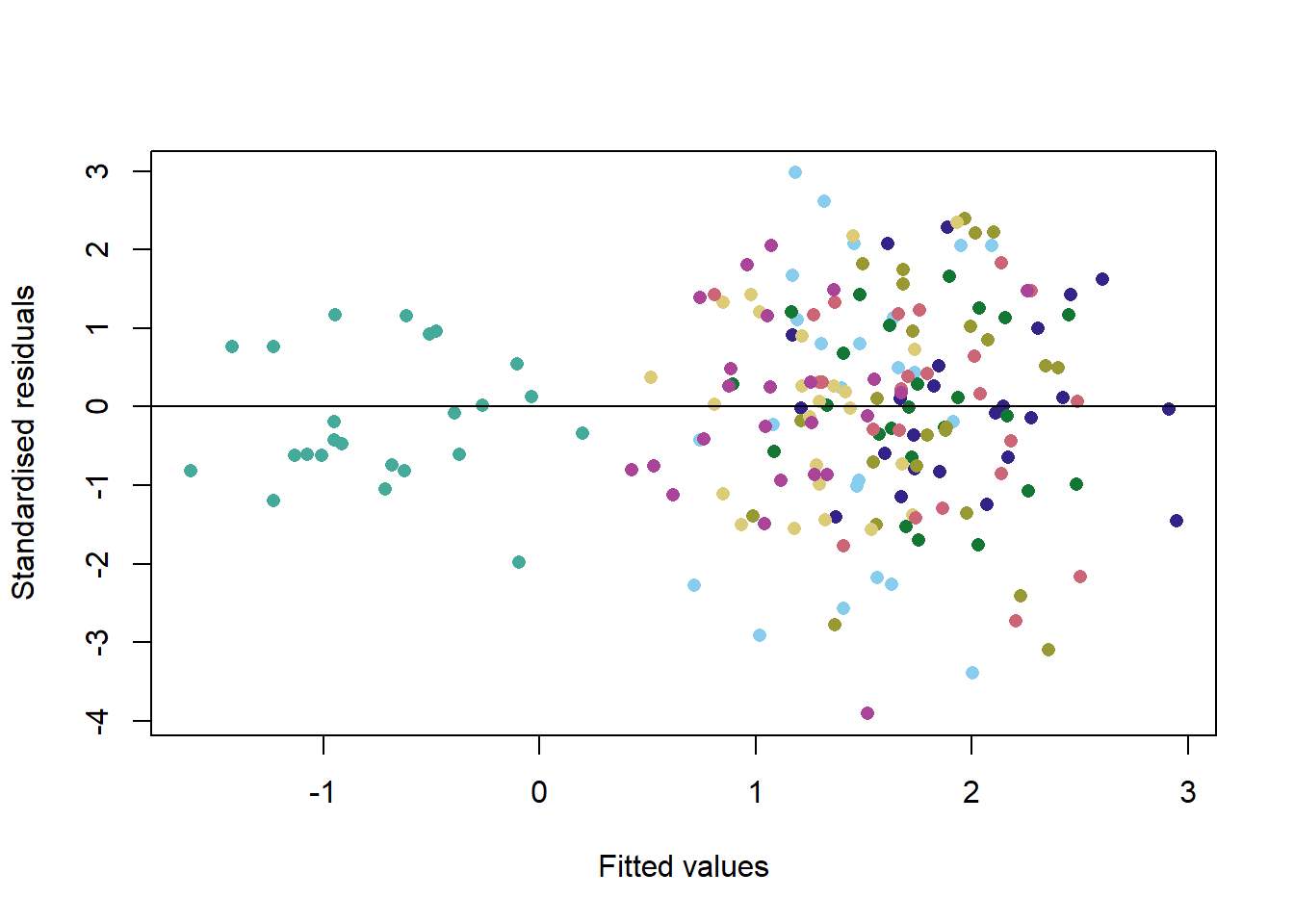#Plotting the residuals against each explanatory variable follows a very similar approach
#In our case we plot standardised residuals against temperature and colour the plots by region
plot(model, resid(., scaled=TRUE) ~ temp, abline = 0,pch=16,col=cols[data\$region],xlab="Temperature",ylab="Standardised residuals")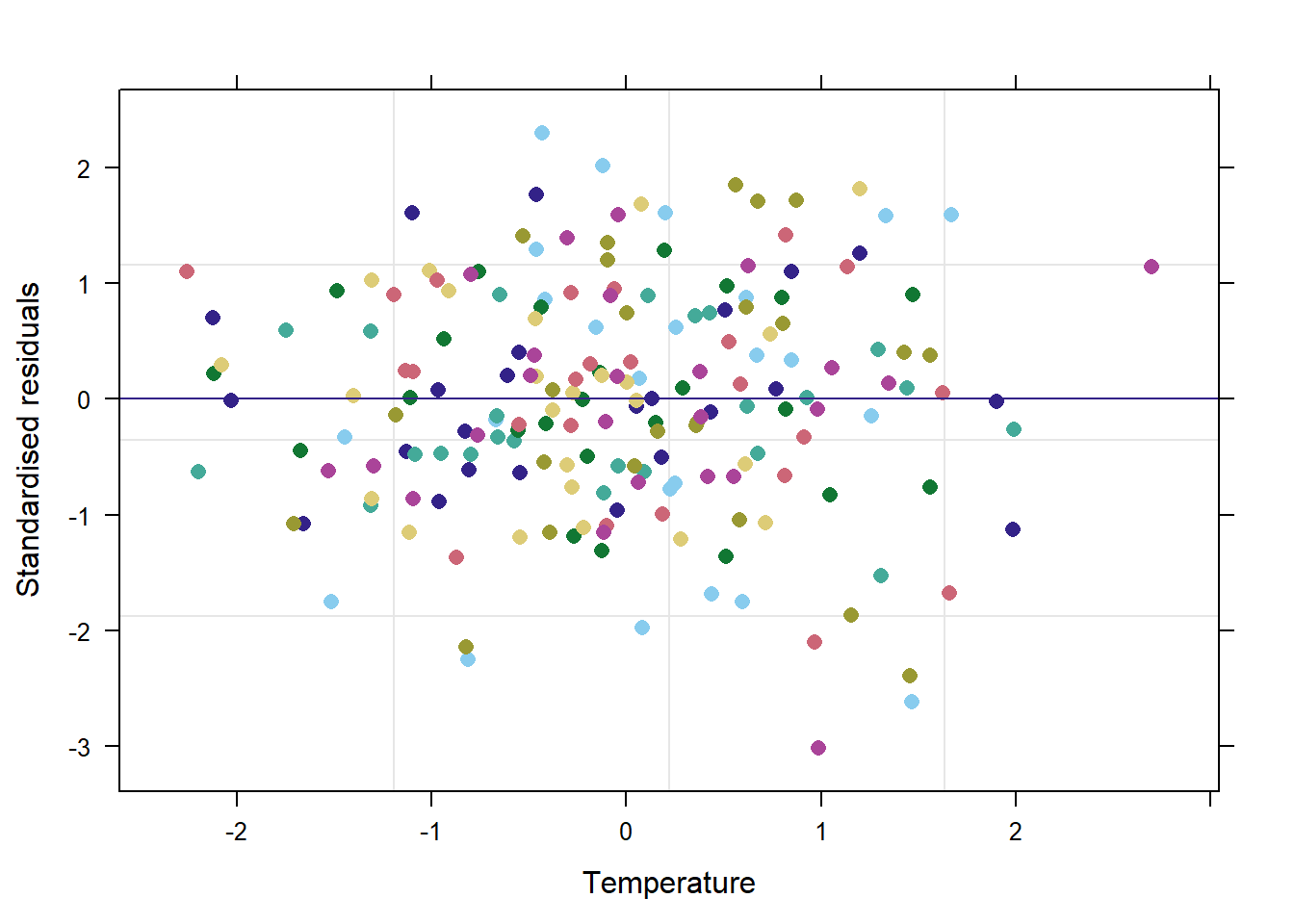Step two. Diagnostic plots of the residuals split by random effect groupings

An additional assumption of mixed effects models is there is not heteroscedasticity among different levels of the random effects. Again, we can check this using plots of the residuals, simply split by levels of the random effect of interest

#We can use a default plot of the standardised residuals versus fitted
#But add | <random effect> to see it per level of the random effect
plot(model, resid(., scaled=TRUE) ~ fitted(.)| region, abline = 0,pch=16,xlab="Fitted values",ylab="Standardised residuals")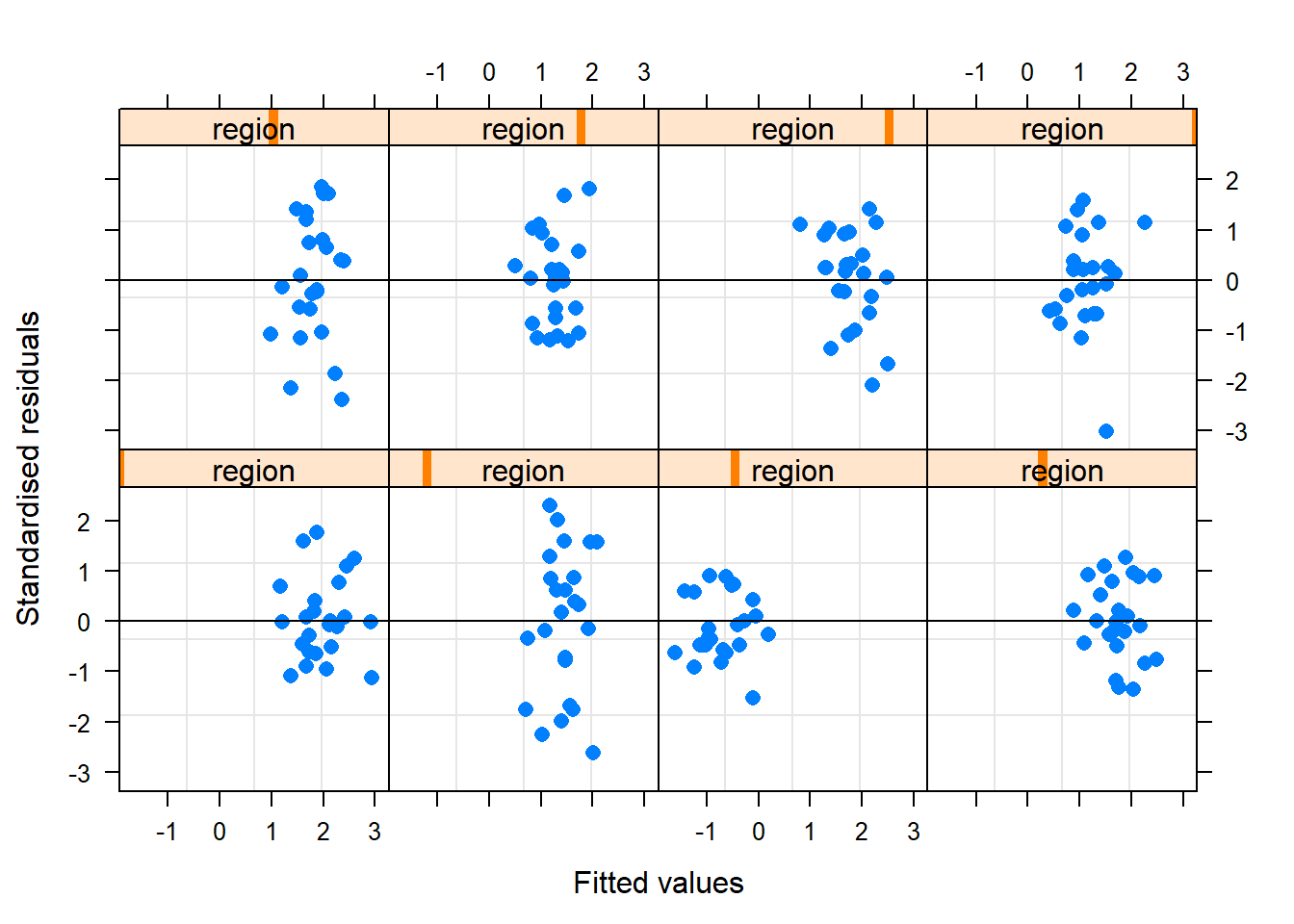#It is also possible to code this manually if greater flexibility is desired
#We provide an example specific to our model here
#You may need to change some of the par and plot arguments for your own models
par(mfrow=c(2,4))
for(i in 1:length(unique(data\$region))){
plot(resid(model,type="pearson")[data\$region==i]~predict(model)[data\$region==i],pch=16,ylim=c(-4,4),main=paste("Region",i),xlab="Fitted values",ylab="Standardised residuals")
lines(x=c(-1000,1000),y=c(0,0))
}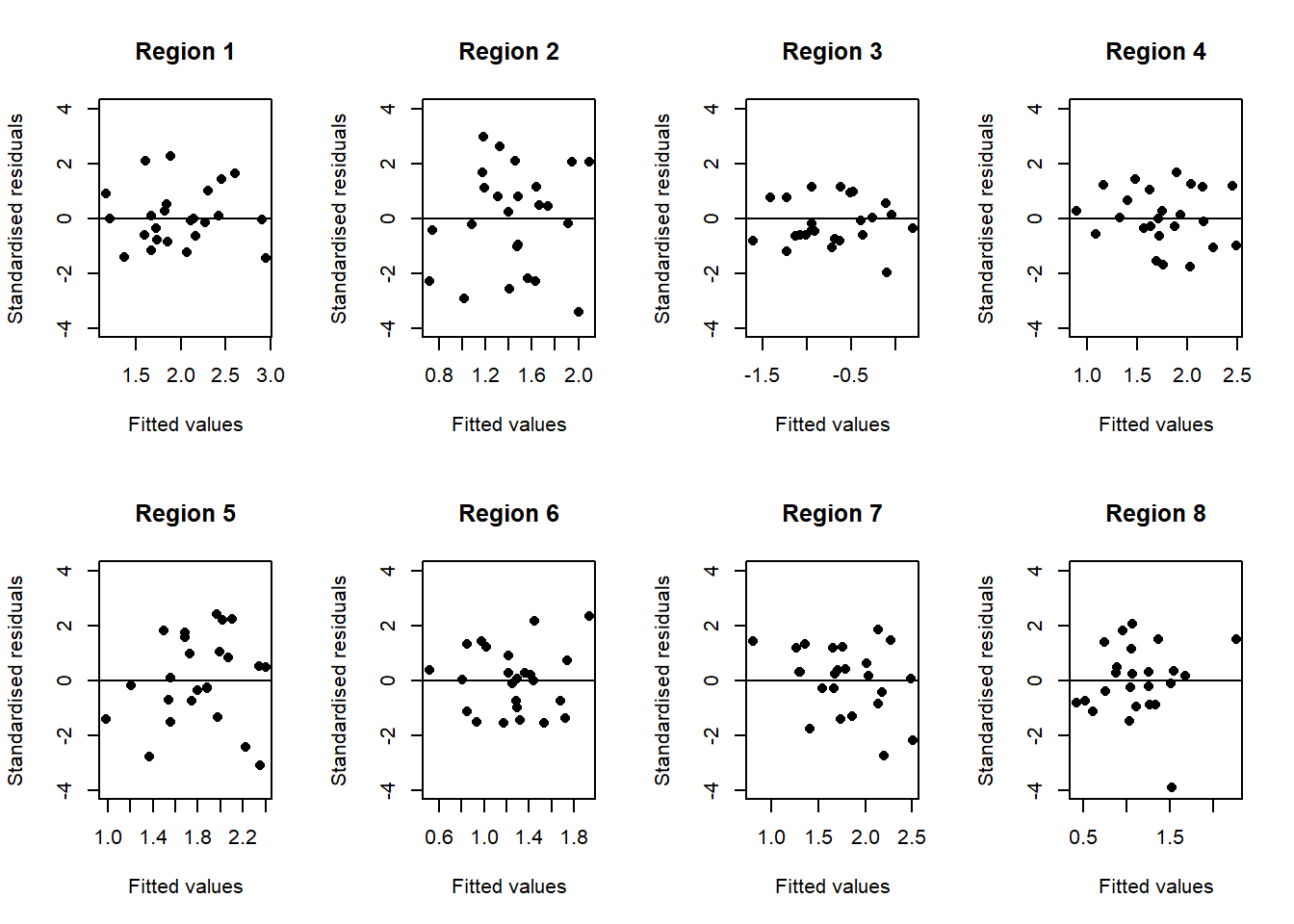par(mfrow=c(1,1))

#You can also compare the difference in residuals between random effect levels using default boxplots
plot(model, as.factor(region) ~ resid(., scaled=TRUE),abline=0,pch=16,xlab="Standardised residuals",ylab="Regions")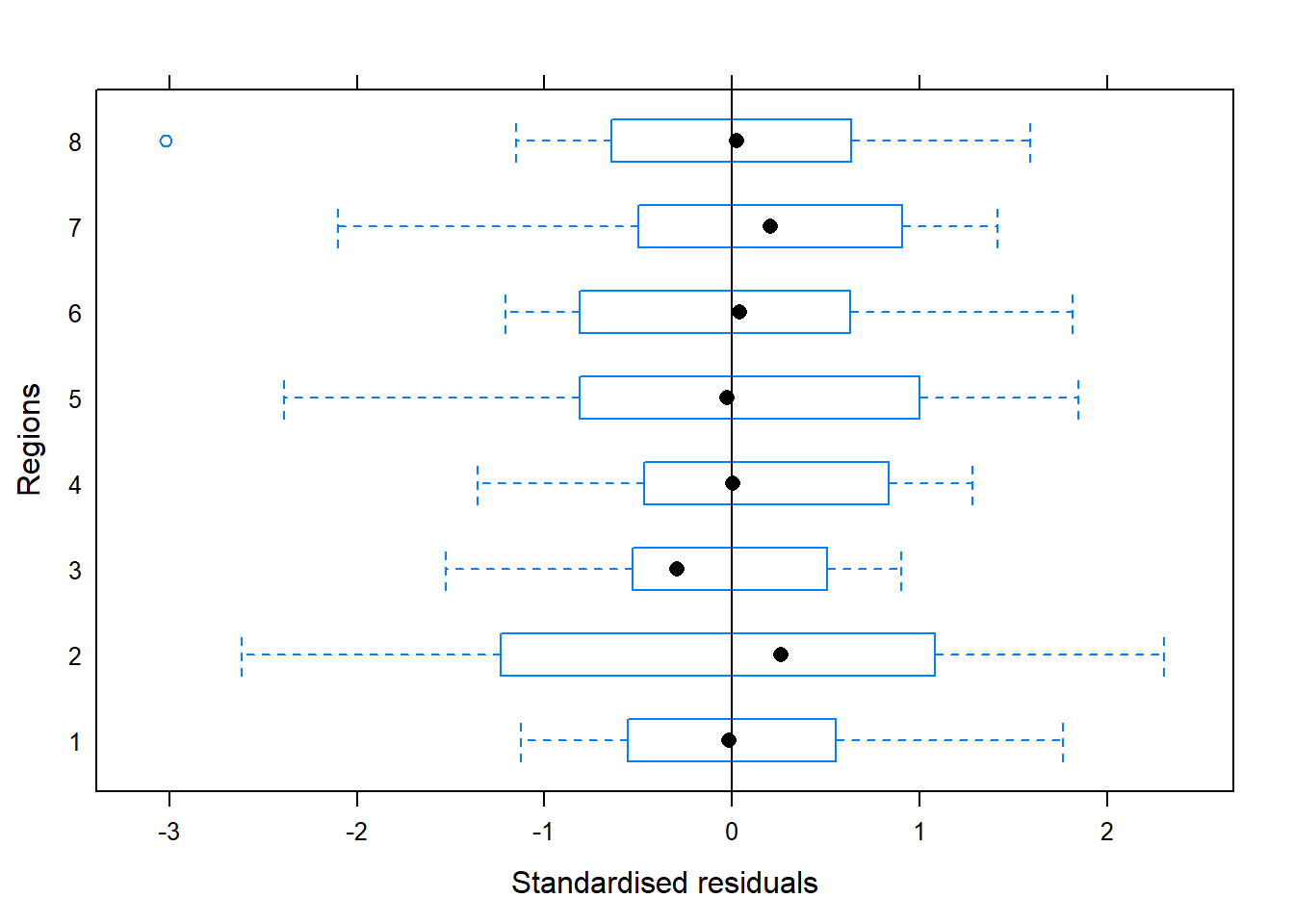Step three. QQ plots

It is also important to check that residuals are normally distributed. The most effective way of doing this is using QQ plots as you would for a linear model

Ideally the points on the QQ plot will lie alongside (or close to) the line that is also plotted. Any substantial deviations from it along its length are an important warning sign that the model residuals do not follow a normal distribution

##QQ plot of model
qqnorm(resid(model),pch=16)
qqline(resid(model))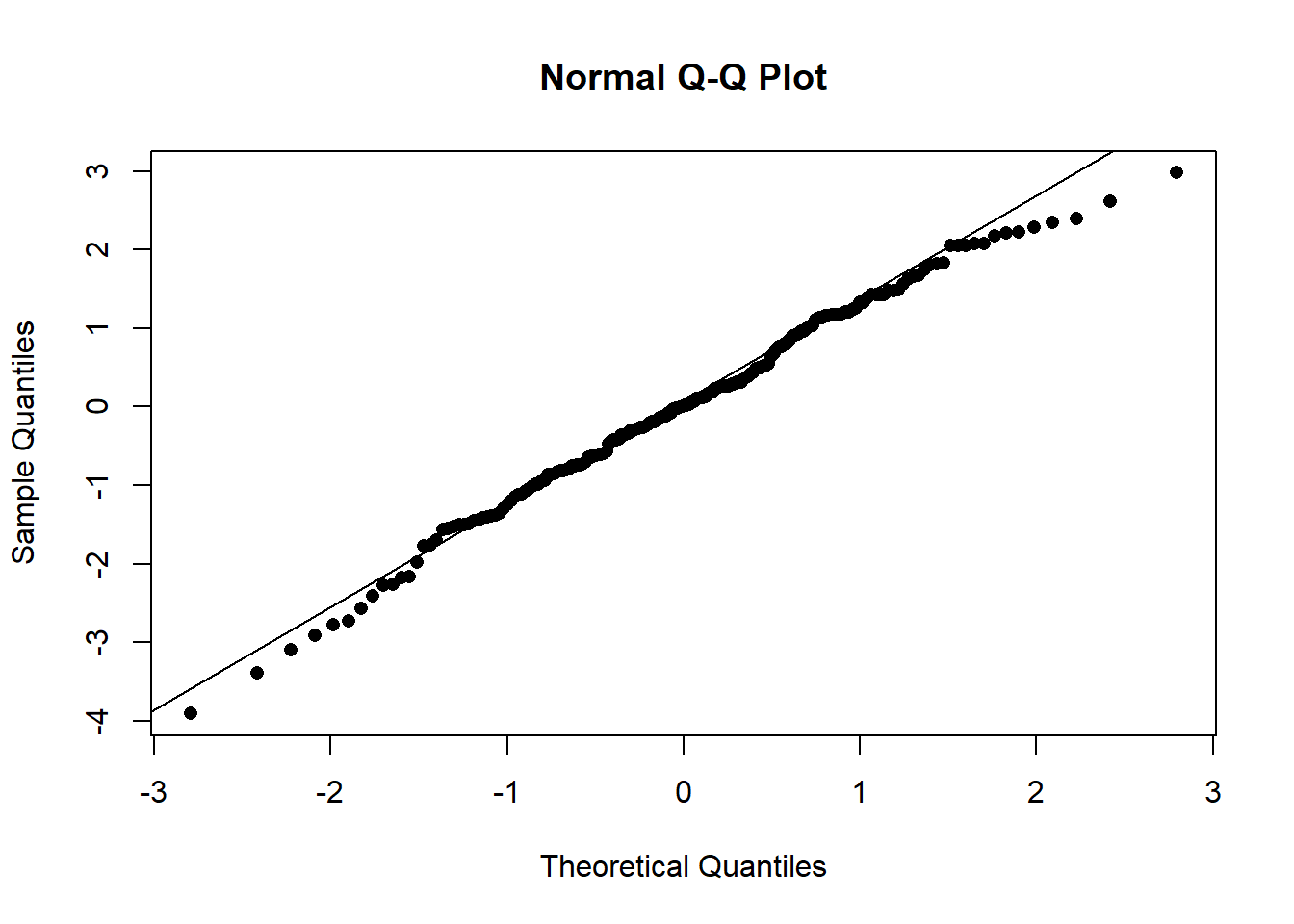##QQ plot of model coloured by region
qqnorm(resid(model),pch=16,col=data\$region)
qqline(resid(model))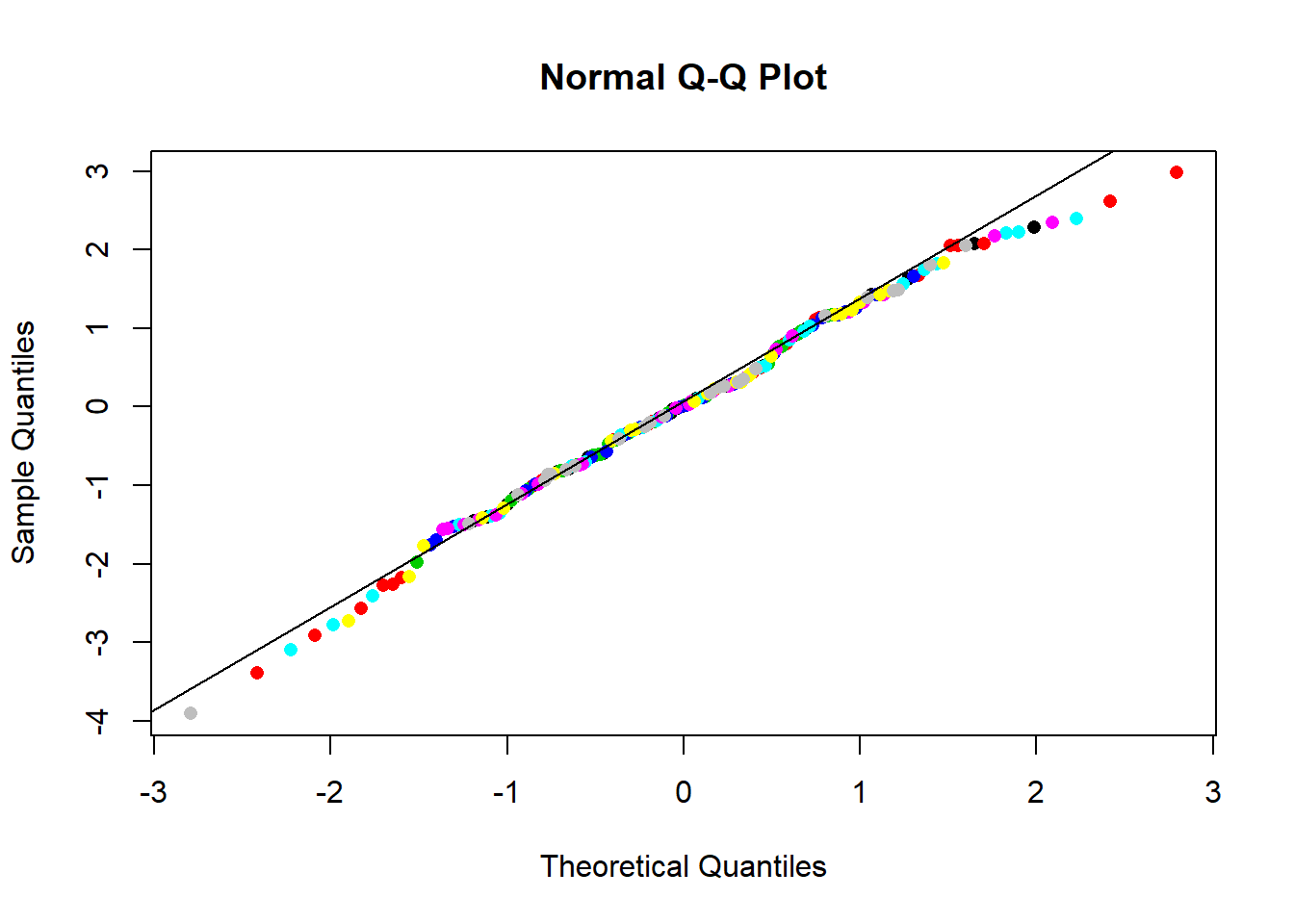Step four. Leverage and Cook’s distance

These measures give a good idea of the influence of particular datapoints on the model, and so make it possible to detect whether the model fit is being driven by outliers in the data

#Calculate leverage
lev<-hat(model.matrix(model))

#Plot leverage against standardised residuals
plot(resid(model,type="pearson")~lev,las=1,ylab="Standardised residuals",xlab="Leverage")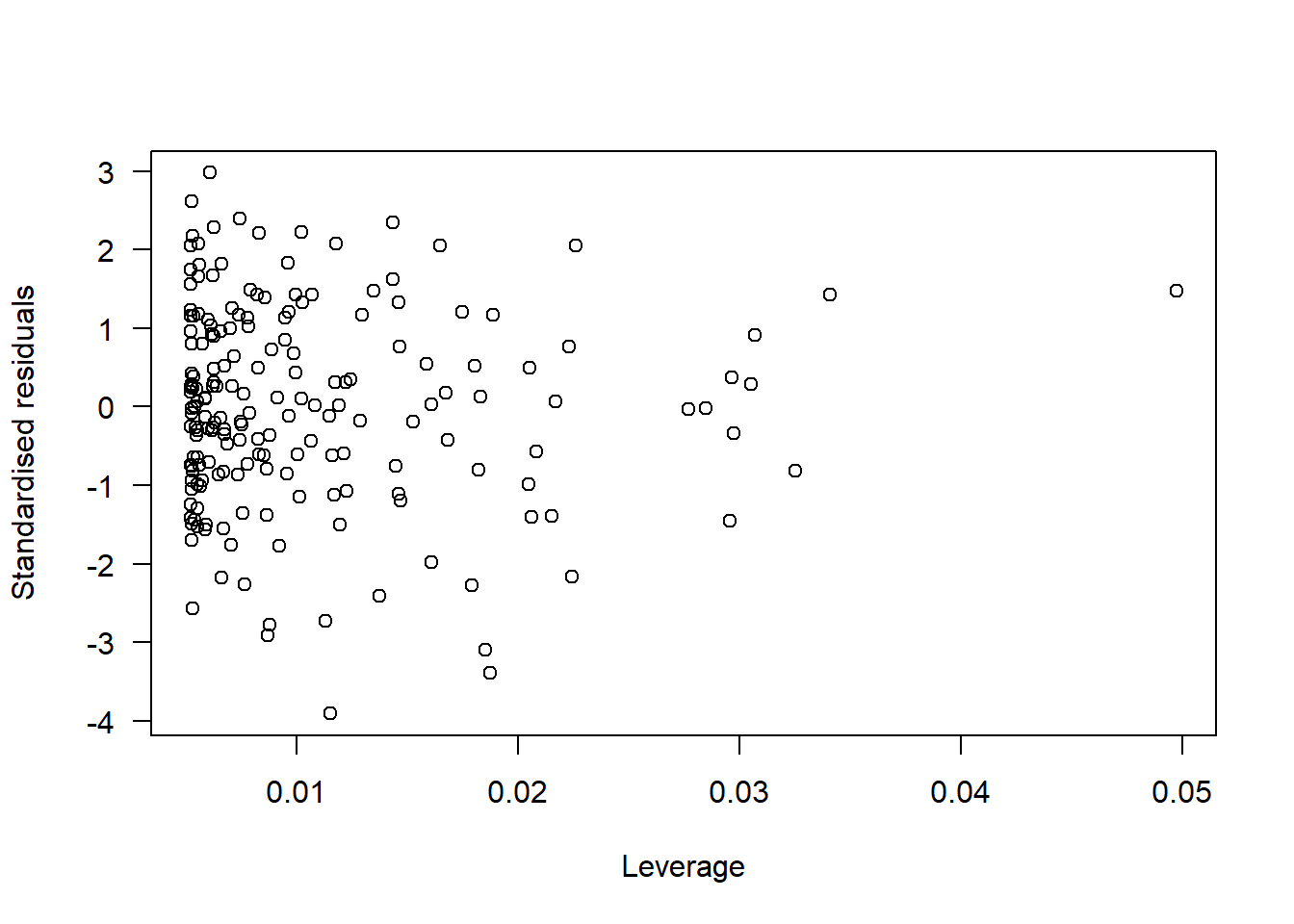#Calculate Cook's Distance
cd<-cooks.distance(model)
#N.B. If Cook's distance is greater than 1 this highlights problematic datapoints

#Plot leverage and Cook's distance together
par(mfrow=c(1,1))
plot(lev,pch=16,col="red",ylim=c(0,1.2),las=1,ylab="Leverage/Cook's distance value")
points(cd,pch=17,col="blue")
points(x=150,y=1.1,pch=16,col="red")
points(x=150,y=0.9,pch=17,col="blue")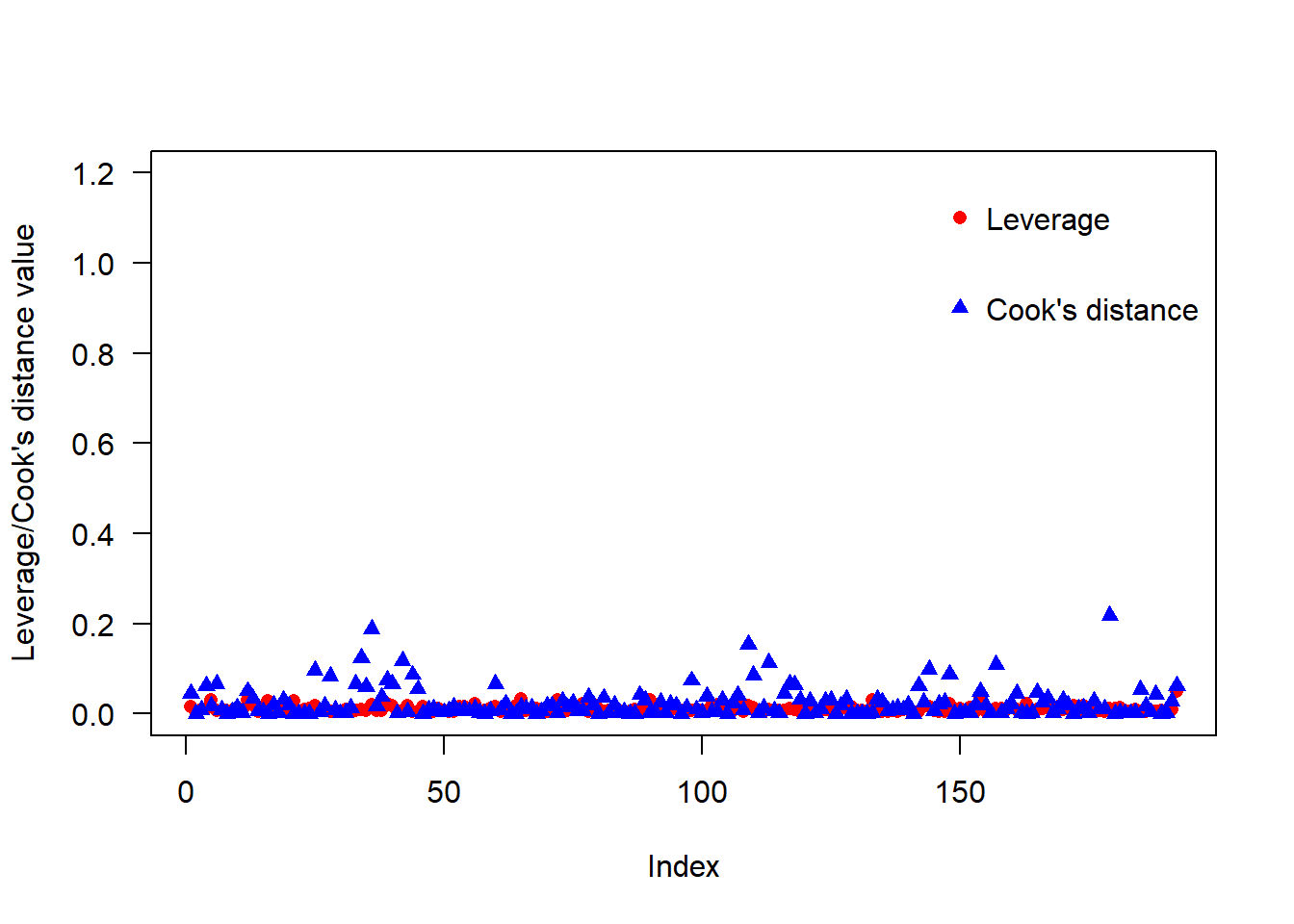Step five. Inspect the random effect distribution

It is also important to check that the random effect distribution(s) match the assumed (most often Gaussian) dsitrbibution

#It is possible to do this using a histogram but this can be unclear if there are few random effect levels as in our example
hist(as.vector(unlist(ranef(model)\$region)),breaks=seq(-2,1,0.25),col=cols,border=NA)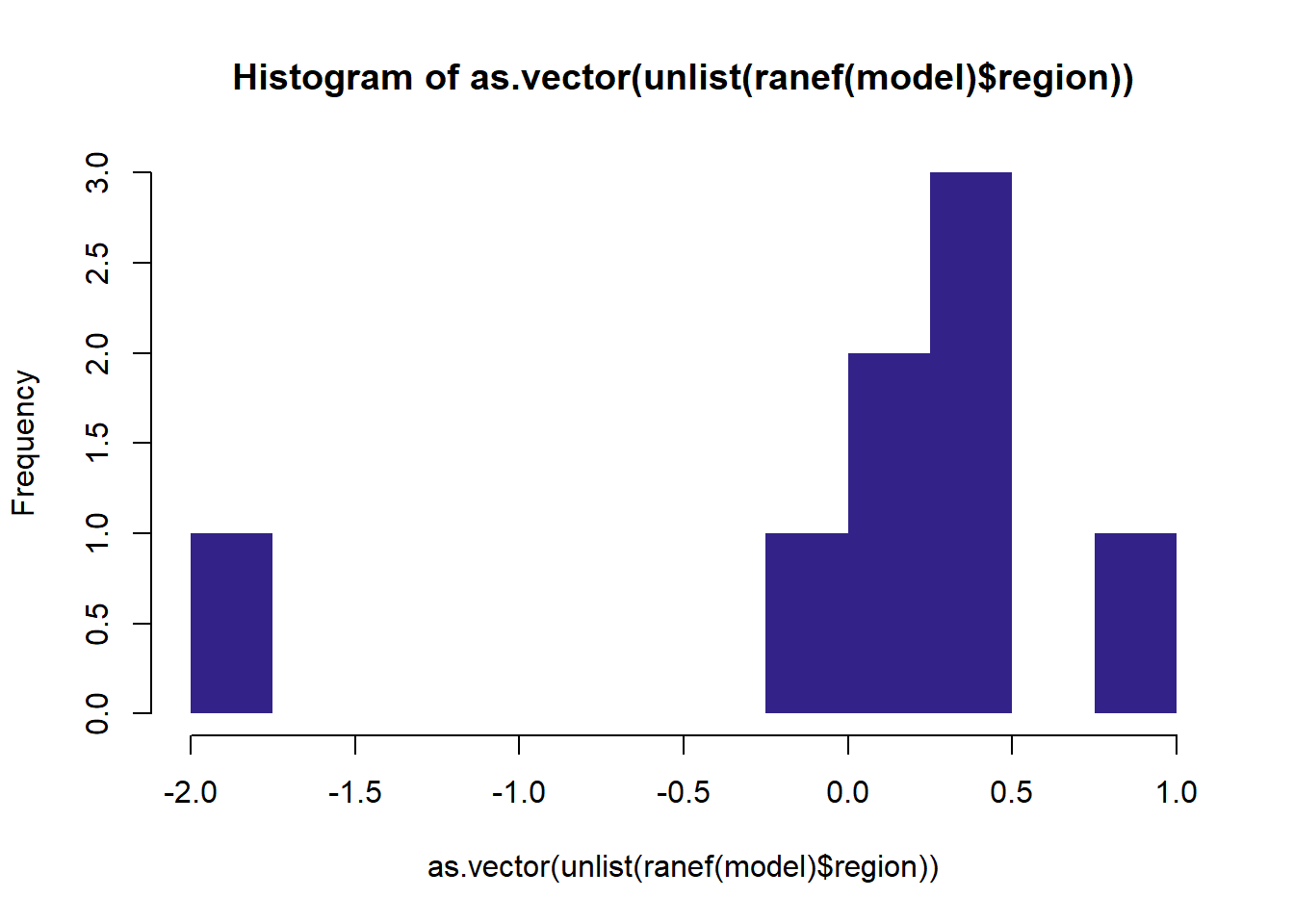#A dotplot is a more effective way of doing this.
lattice::dotplot(ranef(model, condVar=TRUE))
## \$region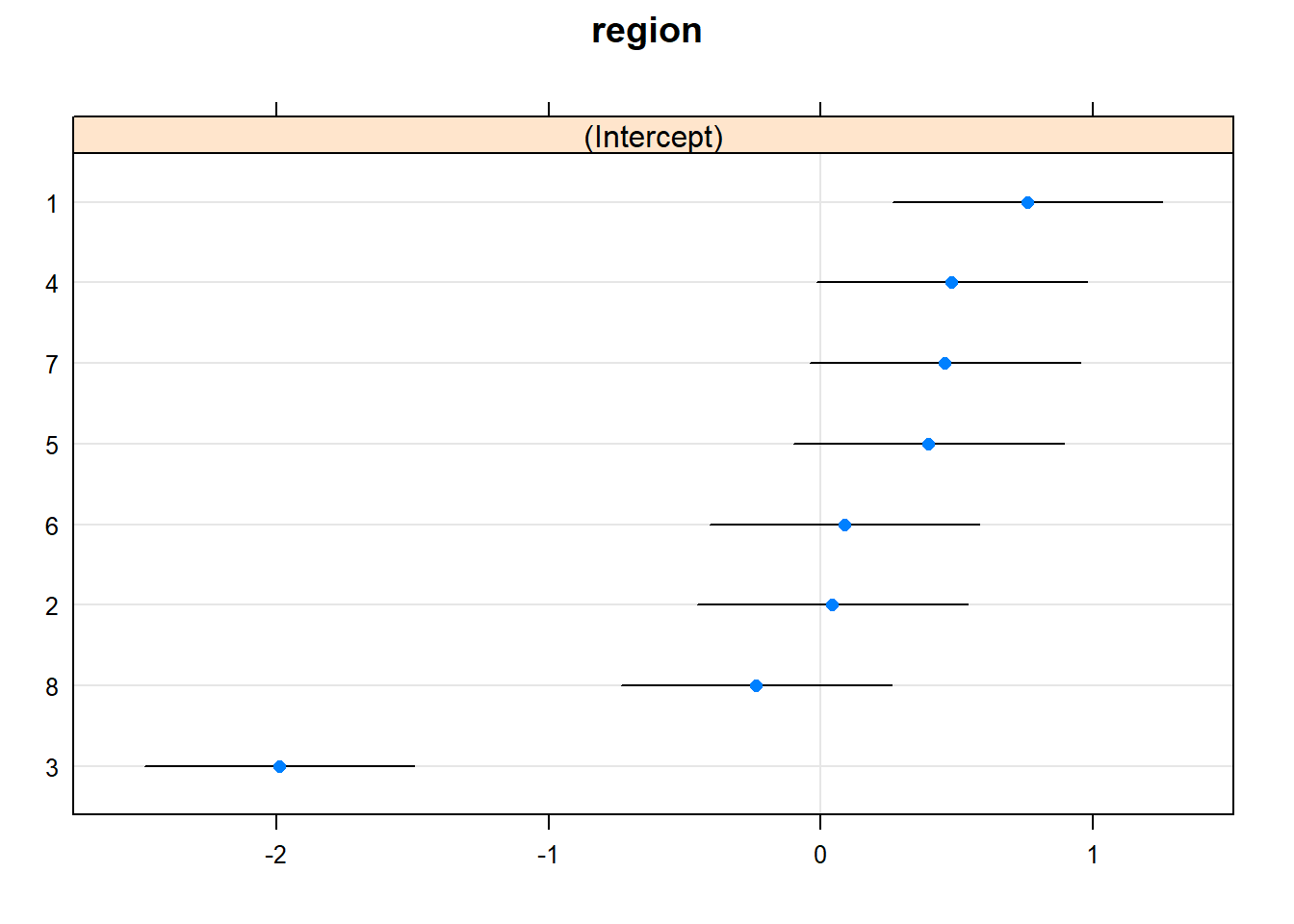#If more flexibility is required it is possible to produce the dotplot manually.
#Note that we calculate 95% confidence intervals in the code that does the plotting
ranefs<-as.data.frame(ranef(model))
ranefs2<-ranefs[order(ranefs\$condval),]

plot(NULL,ylim=c(0,9),xlim=c(-2.5,1.5),yaxt="n",ylab="Random effect level",xlab="",cex.axis=1.5,cex.lab=1.5)
lines(x=c(0,0),y=c(-1,10))
for(i in 1:nrow(ranefs2)){
lines(x=c(ranefs2[i,4]-ranefs2[i,5]*1.96,ranefs2[i,4]+ranefs2[i,5]*1.96),y=rep(i,2))
points(x=ranefs2[i,4],y=i,cex=1.5,pch=21,col="dark blue",bg="dodger blue")
}
axis(side=2,at=seq(1,8,1),labels=ranefs2\$grp,las=1,cex.axis=1.5)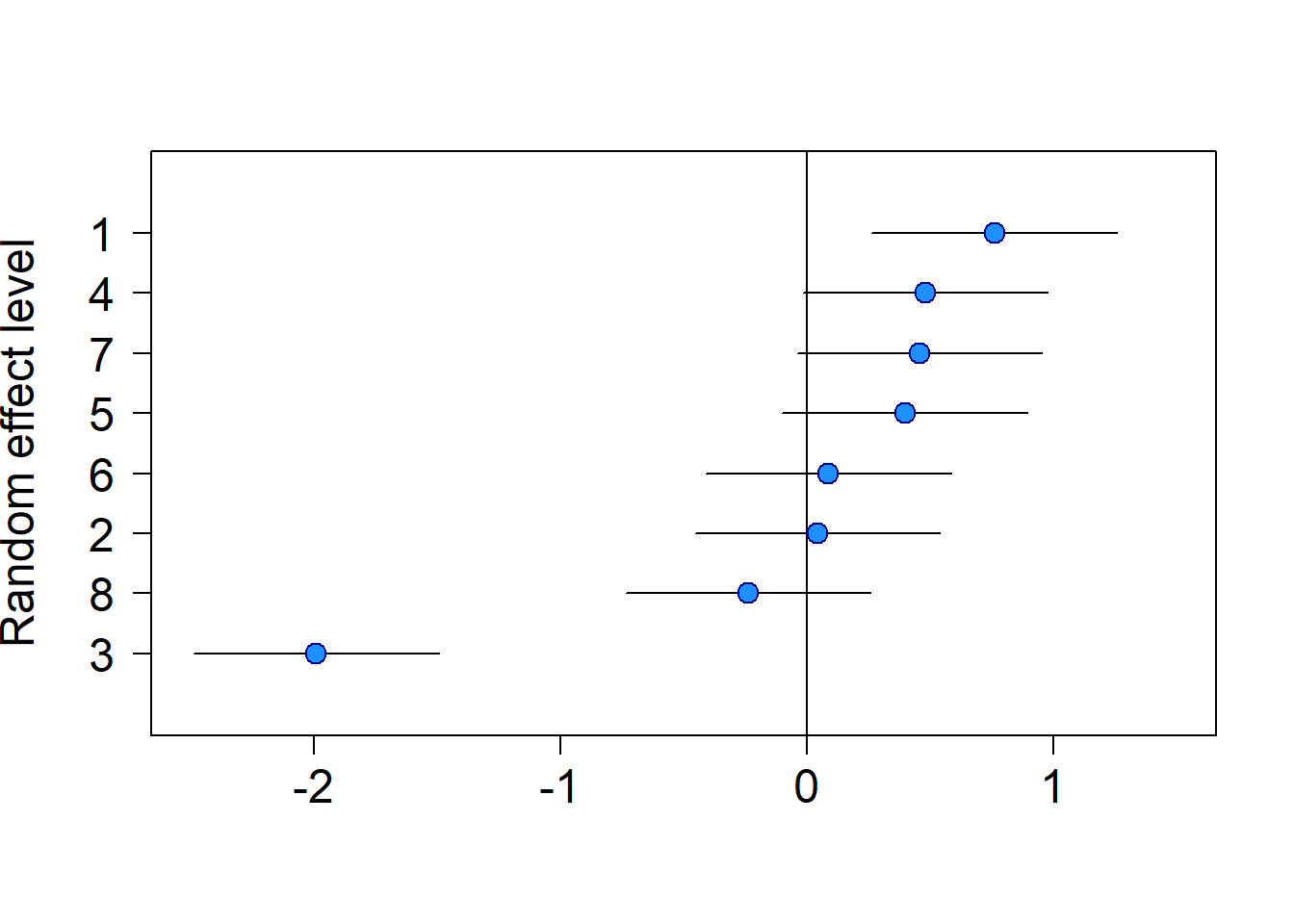Step six. Checking for spatial autocorrelation

In many situations in ecology it might also be important to test for autocorrelation (spatial/temporal etc.). The easiest option is to refit models in nlme. However, this is not useful for GLMMs (nlme will only fit Gaussian models). It is also possible to calculate semi-variograms yourself using the model residuals and spatial data in the dataset.

First, the nlme approach. We show three semi-variograms here: 1. The semi-variogram for the linear model between temperature and height (fitted using generalised least squares) 2. The semi-variogram for the LMM with region as a random effect 3. The semi-variogram for the LMM with site as a random effect

It is possible to see from these that Region handles some of the autocorrelation, but that Site does a much better job

#1. Linear model
sp_model<-gls(height~temp,data=data)
plot(Variogram(sp_model, form= ~ x + y))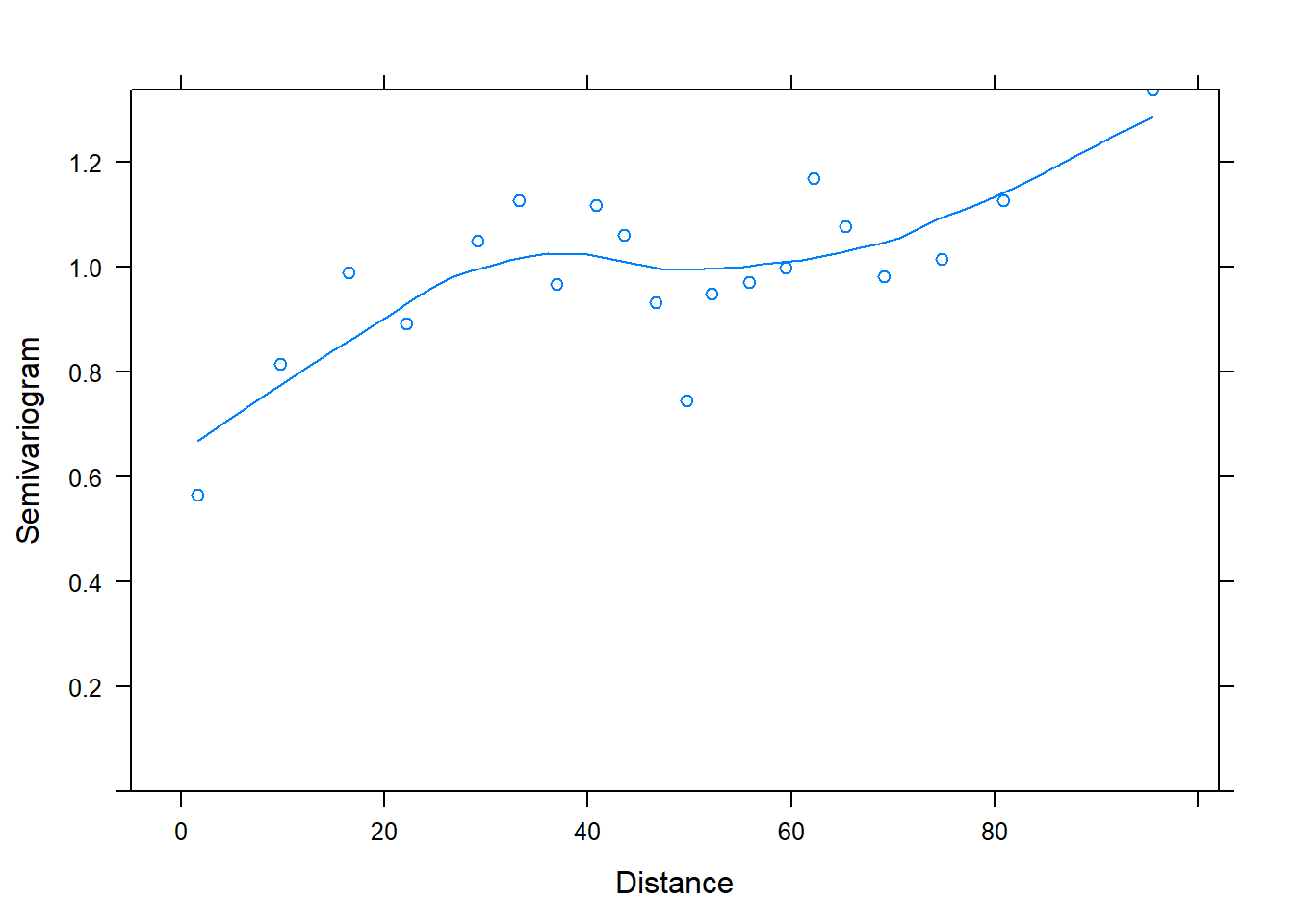#2. Region as a random effect
sp_model2<-lme(height~temp,random=~1|region,data=data)
plot(Variogram(sp_model2, form= ~ x + y))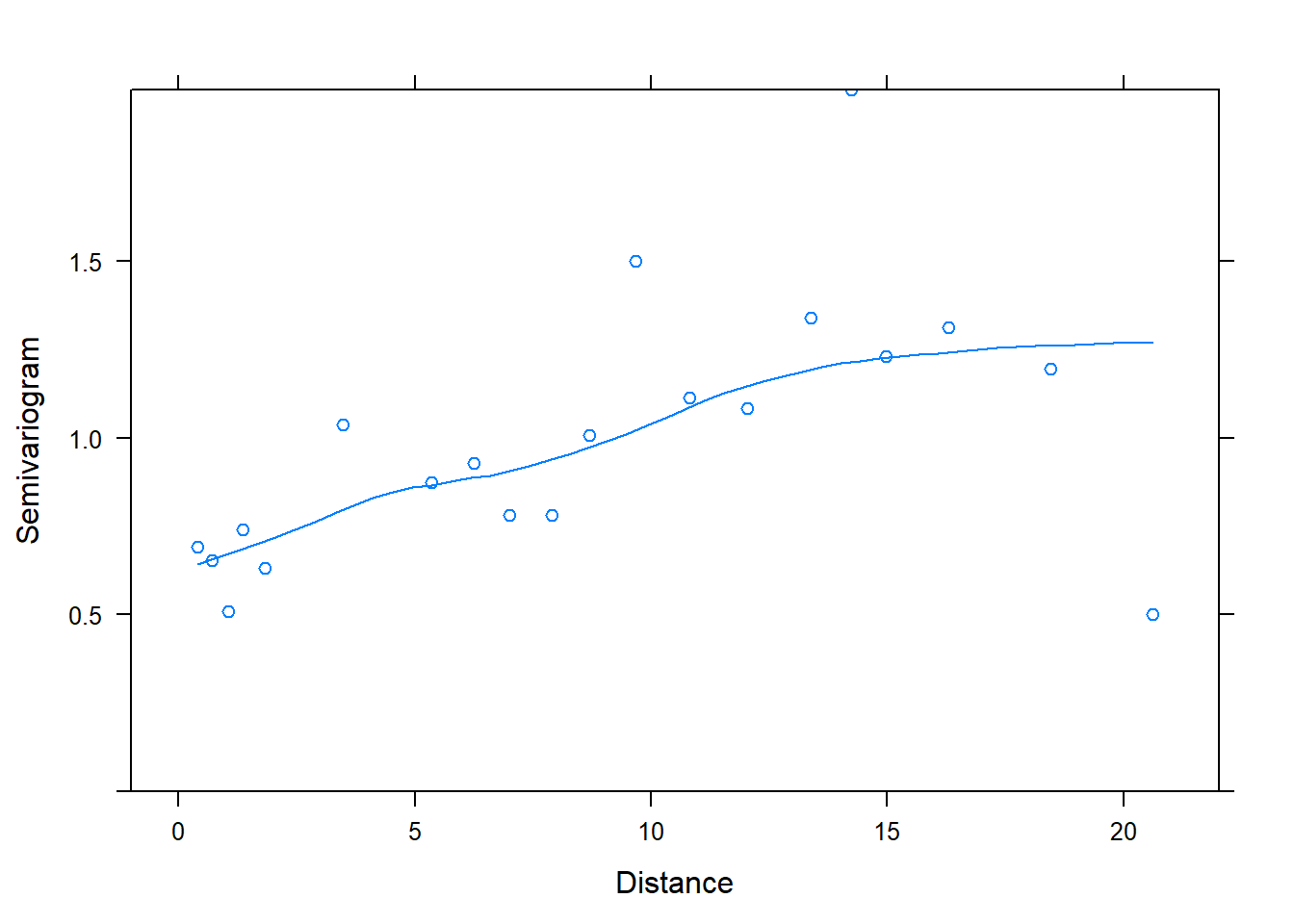#3. Site as a random effect
sp_model3<-lme(height~temp,random=~1|site,data=data)
plot(Variogram(sp_model3, form= ~ x + y))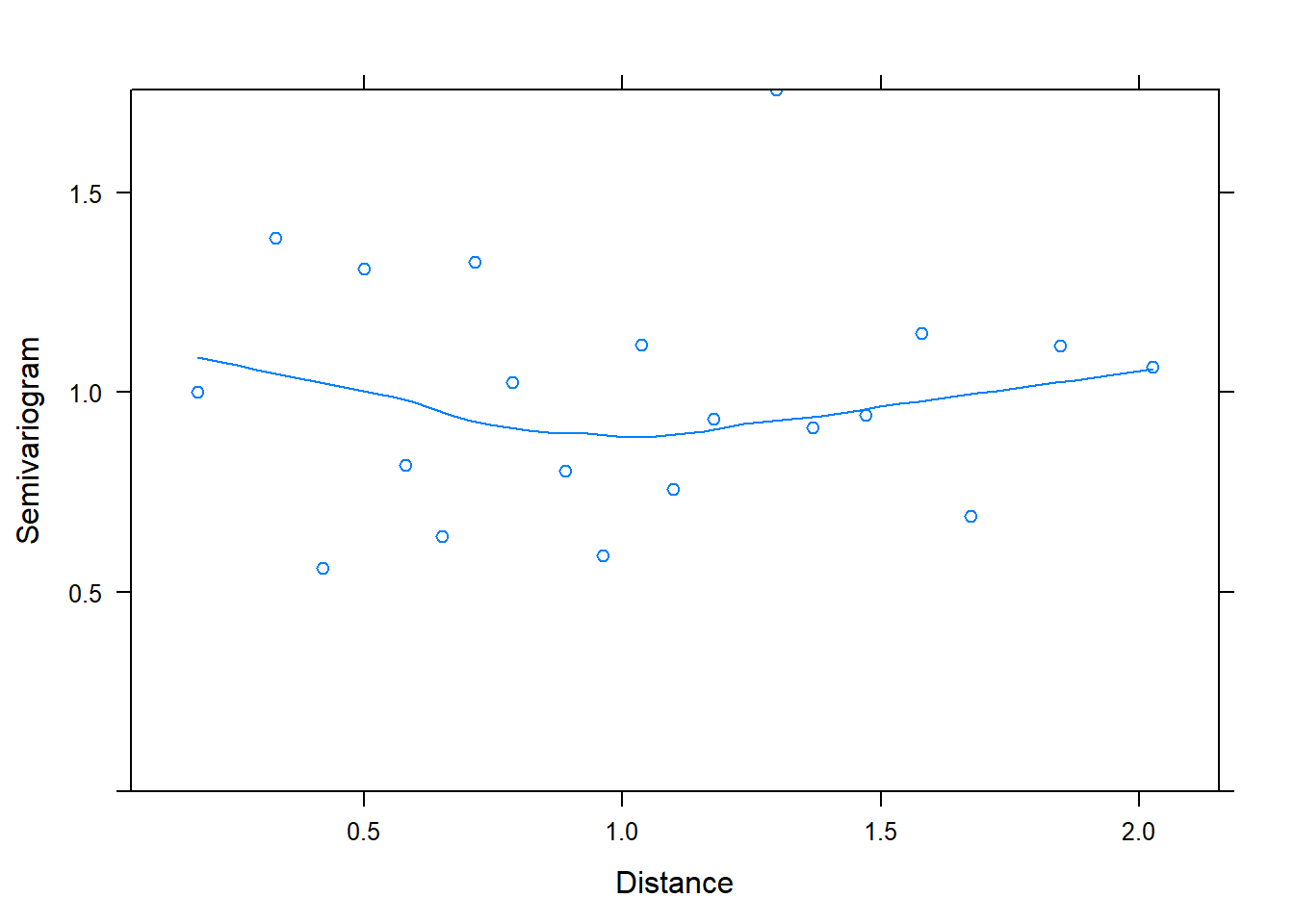We now use the variog() function in the geoR package to fit our own semi-variograms using the model residuals and the x and y columns from the dataset.

By taking this approach we add a bit more fine-scale detail to our semi-variogram. We can see that while including Site as a random effect completely deals with spatial autocorrelation, using region leads to some strange spatial patternings in the data (while reducing spatial autocorrelation relative to the case where no random effect is fitted)

#1. Linear model
Bn<-variog(coords=cbind(data\$x,data\$y),data=resid(model.n,type="pearson"),option="bin",breaks=seq(0,50,1))
## variog: computing omnidirectional variogram
plot(Bn)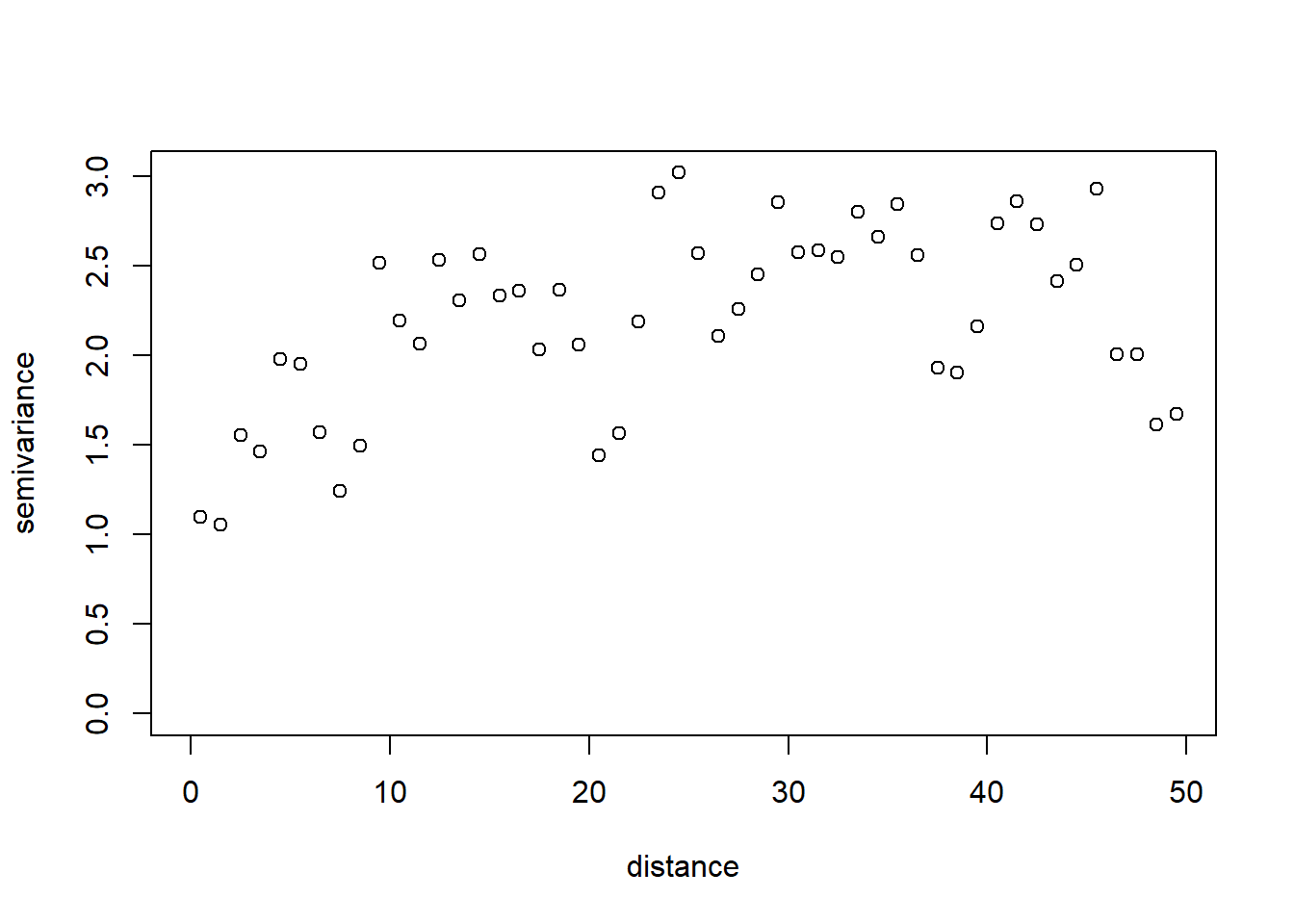#2. Region as a random effect
B<-variog(coords=cbind(data\$x,data\$y),data=resid(model,type="pearson"),option="bin",breaks=seq(0,50,1))
## variog: computing omnidirectional variogram
plot(B)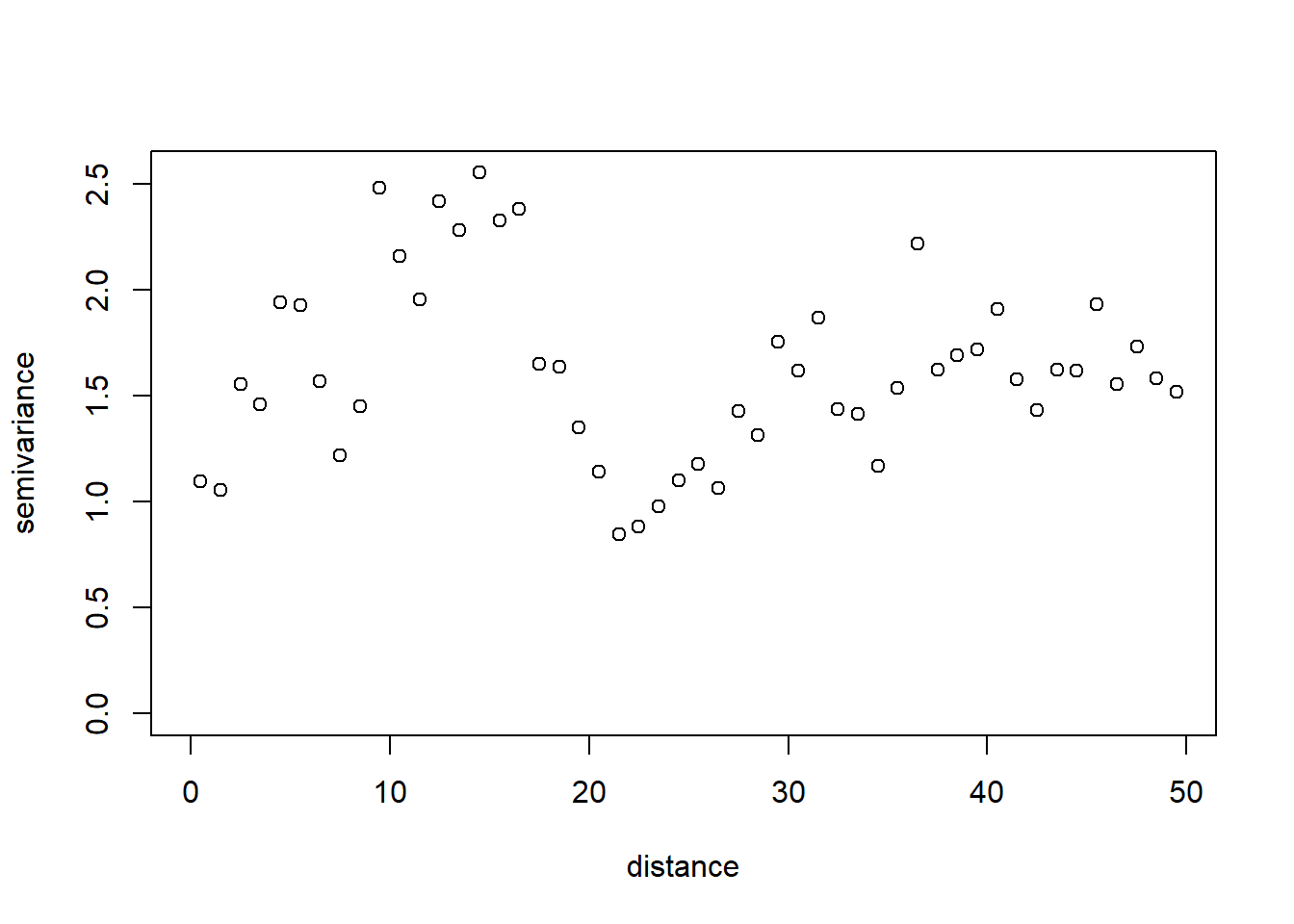#3. Site as a random effect
B2<-variog(coords=cbind(data\$x,data\$y),data=resid(model2,type="pearson"),option="bin",breaks=seq(0,50,1))
## variog: computing omnidirectional variogram
plot(B2)